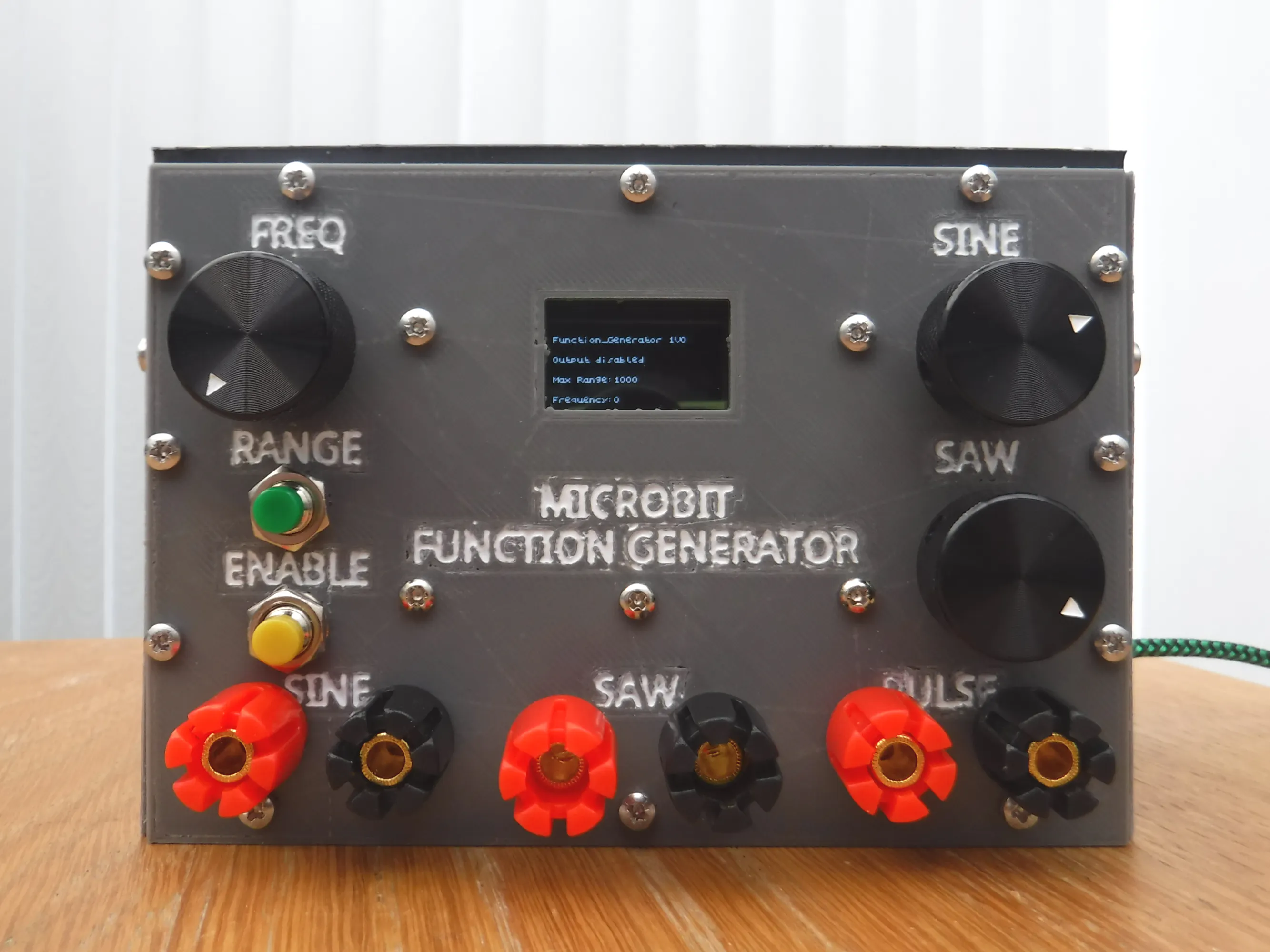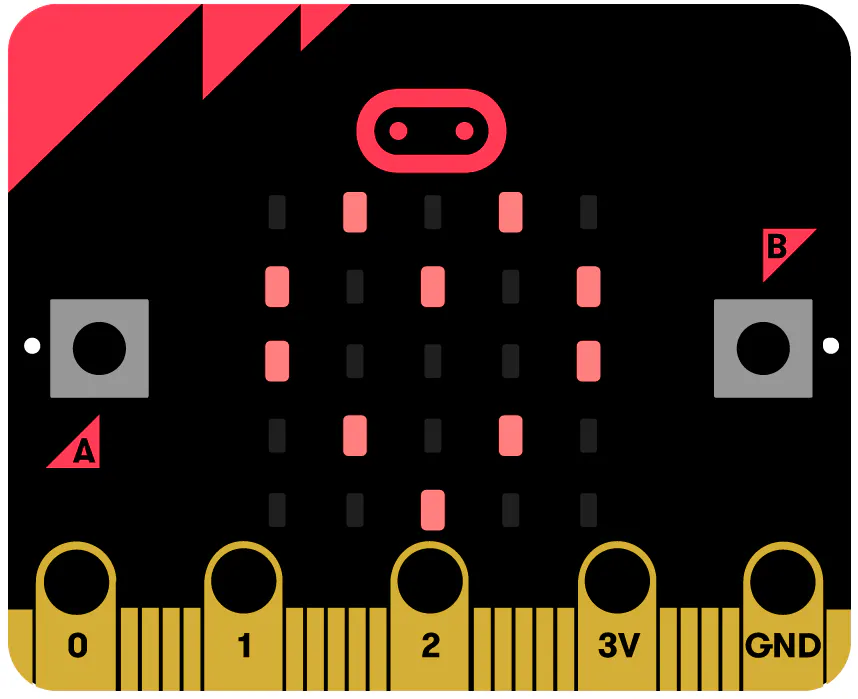# Microbit Function Generator

A Function generator producing sine, sawtooth and pulses up to 1MHz using a Microbit, custom PCB and 3d printed box.## Things used in this project

### Hardware componentsBBC micro:bit board
×1
 OLED Display
×1Test Accessory, Dual Banana Jack to Male BNC Adapter Optional connections
×3
 5K Potentiometer
×2
 100k Multiturn Potentiometer
×1
 Control Knobs 20 x 15.5 mm, ~6mm diameter spindle Subject to personal choice and potentiometer selection.
×3
 Microbit breakout board
×1
 Jumper wires M/F
×23
 Resistor 15k
×3
 Resistor 30k
×3
 Resistor 60k
×3
 Resistor 120k
×3
 Resistor 100k
×1
 Resistor 200R
×2
 Resistor 150R
×1
 Resistor 330R
×1
 Capacitor 100nF
×6
 Capacitor 100uF/10V
×1
 CD4024 - 7 stage Binary Counter
×1
 CD4015 - Dual shift register
×1
 CD4070 - Quad 2 input EXOR
×1
 MCP602 - Dual Op-Amp
×1
 Terminal Block - 2 pin 5mm pitch
×1
 M3 8mm screw
×32
 M3 10mm screw
×1
 M3 30mm screw
×2

### Software apps and online services

 Microbit makecode
 Slicer Cura

### Hand tools and fabrication machines3D Printer (generic)Soldering iron (generic)Wire Stripper & Cutter, 26-16 AWG / 0.4-1.29mm Capacity Wires

## Custom parts and enclosures

### Enclosure STL files

Files required to 3d printing the side panela

## Schematics

D2A converter

### PCB gerber files

Files to enable PCB creation

## Code

### microbit_VPEN_VIEW_JS.txt

JavaScript
Microbit code for Function Generator (Makecode conversion)
```function centre () {
if (o_enable == 0) {
led.unplot(2, 2)
led.plot(4, 4 - range)
} else {
led.plot(2, 2)
led.plot(4, 4 - range)
}
}
input.onButtonPressed(Button.A, function () {
if (o_enable == 0) {
o_enable = 1
old = 0
led.plot(2, 2)
} else {
o_enable = 0
led.unplot(2, 2)
}
if (o_enable == 0) {
kitronik_VIEW128x64.show("Output disabled", 3)
} else {
kitronik_VIEW128x64.show("Output enabled", 3)
}
})
freq = 0
pct = (bin - 1) / 1023 + offset[range]
if (pct > 0) {
freq = max_range * pct
}
if (old > bin - 20 && old < bin + 20) {
clr_scrn()
for (let index2 = 0; index2 <= 9 * (pct * 1.25); index2++) {
indx2 = -4 + index2
for (let index = 0; index <= 4; index++) {

}
}
kitronik_VIEW128x64.show("Frequency:" + Math.trunc(freq) + "  ", 7)
}
old = bin
centre()
}
function clr_scrn () {
for (let index2 = 0; index2 <= 9 * (1 * 1.25); index2++) {
indx2 = -4 + index2
for (let index = 0; index <= 4; index++) {
led.unplot(index, index - indx2)
}
}
centre()
}
input.onButtonPressed(Button.B, function () {
range += 1
if (range > list.length - 1) {
range = 0
led.unplot(4, 0)
led.plot(4, 4)
} else {
led.unplot(4, 4 - (range - 1))
led.plot(4, 4 - range)
}
max_range = list[range]
kitronik_VIEW128x64.show("Max Range:" + list[range] + "  ", 5)
})
function reset () {
list = [
1000,
10000,
100000,
250000,
500000
]
max_range = list
offset = [
0.007,
0.0069,
0.00685,
0.006844,
0.005906
]
xval = 0
yval = -1
value = -1
xsig = 3
ysig = -1
scale = -1
freq = 0
range = 0
multiplier = 0
o_enable = 0
pins.analogPitch(0, 0)
music.ringTone(0)
}
let multiplier = 0
let scale = 0
let ysig = 0
let xsig = 0
let value = 0
let yval = 0
let xval = 0
let indx2 = 0
let max_range = 0
let offset: number[] = []
let pct = 0
let bin = 0
let old = 0
let range = 0
let o_enable = 0
let freq = 0
let list: number[] = []
pins.analogSetPitchPin(AnalogPin.P0)
music.setBuiltInSpeakerEnabled(false)
basic.showString("F_GEN")
reset()
led.plot(4, 4)
kitronik_VIEW128x64.controlDisplayOnOff(kitronik_VIEW128x64.onOff(true))
kitronik_VIEW128x64.show("Function_Generator 1V0", 1)
kitronik_VIEW128x64.show("Output disabled", 3)
kitronik_VIEW128x64.show("Max Range:" + list, 5)
kitronik_VIEW128x64.show("Frequency:" + Math.trunc(freq), 7)
basic.forever(function () {
if (o_enable == 1) {
if (freq >= 1) {
music.ringTone(freq)
} else {
music.ringTone(0)
}
} else {
music.ringTone(0)
}
})
```

## Credits

### C Forde

7 projects • 1 follower